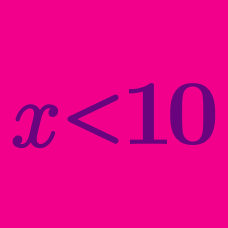Algebra

# Linear Inequalities Warmup

If $x < -3$ and $y > 1,$ the value of $xy$ is:

If $x < y$ and $z < x,$ place $x, y,$ and $z$ in order from least to greatest value.

True or False?

If $-x \leq -7,$ then $x \leq 7.$

Given the following inequalities, what could be true about $x$ and $y$? $x + y < z$ $x - y > z$ $z > 0$ $y < x$

How many solutions exist for this inequality?

$3x > 12$

×